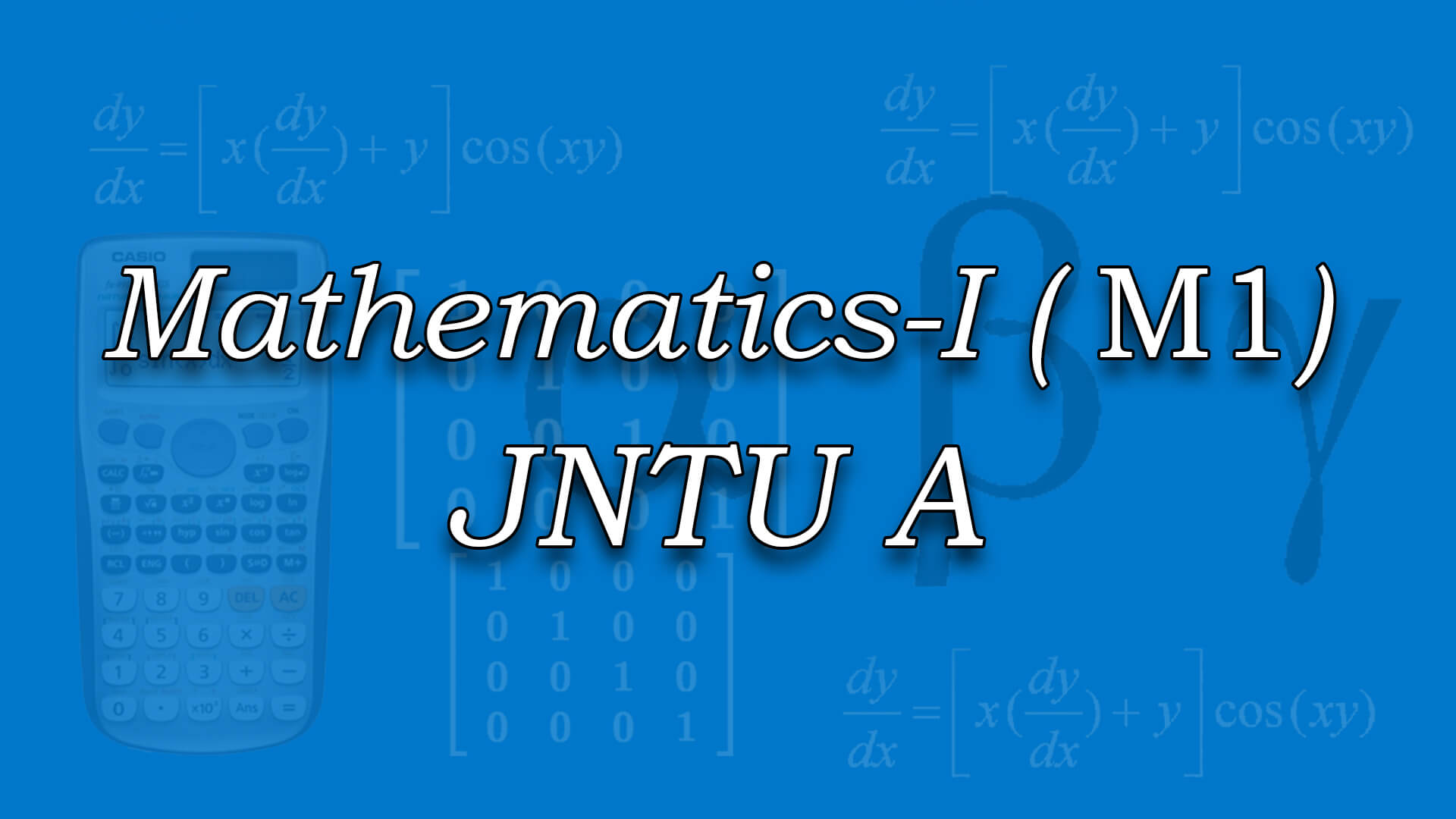## Mathematics-I for JNTUA by Dr C N B Rao ., M.Sc ,Ph.D (IIT Kharagpur ) , Mr C Srinivas MSc ,B.Ed (Osmania) The course is designed to equip the students with the necessary mathematical skills and techniques that are essential for an engineering course. The skills derived from the course will help the student from a necessary base to develop analytic and design concepts. Code : M1JNTUA Recommended For : B.Tech 1st Year JNTUA

#### After Learning this subject,you should be able to

• Mathematical concepts of ordinary differential equations and their applications.
• concepts of differential and Integral calculus, ordinary differential equations and vector calculus.
• Calculate Jacobian and minima of functions of two variables.

## Curriculum 09:12:04   units   |   5

• 3:32:49

UNIT 1
Unit 1: Exact differential equations, Reducible to exact Part 1, Reducible to exact Part 2, Linear & Bernoulli Equations, Linear differential equations of higher order with constant coefficients, Linear differential equations of higher order with constant coefficients - Part 1, Non homogeneous terms with RHS term of the type ax e , sin ax, cos ax 01, Non homogeneous terms with RHS term of the type ax e , sin ax, cos ax,02, Higher order differentional equations Problem 1, Higher order differentional equations Problem 2, Higher order differentional equations Problem 3, Higher order differentional equations Problem 4, Higher order differentional equations Problem 5, Higher order differentional equations Problem 6, Higher order differentional equations Problem 7, Higher order differentional equations Problem 8, Higher order differentional equations Problem 9, Higher order differentional equations Problem 10, Applications : Newton?s law of cooling and natural growth and decay, Problems on Newton?s law of cooling, Problems on natural growth and decay, Orthogonal trajectories, Problems on Orthogonal Trajectories Part 1, Problems on Orthogonal Trajectories Part 2, Problems on EDE, EDE Polar Form, Electrical Circuits Applications

• 1:23:49

UNIT 2
Unit 2: Taylor's Series and Maclaurin's Series, Taylor's Series and Maclaurin's Series Part 1, Taylor's Series and Maclaurin's Series Part 2, Jacobian Determinant, Jacobian Problem 1, Jacobian Problem 2, Functional Dependence and Independence, Maxima and minima of functions of 1 & 2 variables, Lagranges Method, Max and min of functions of 2 & 3 variables using method of Lagrange Multipliers Part 1, Max and min of functions of 2 & 3 variables using method of Lagrange Multipliers Part 2, Max and min of functions of 2 & 3 variables using method of Lagrange Multipliers Part 3

• 1:40:55

UNIT 3
Unit 3: Introduction, Double Integrals, Triple Integrals, Triple Integrals Part 1, Change of variables, Change of order of integration, Applications to find Areas, Applications to find Areas Part 1, Moment of Inertia and volumes, Moment of Inertia and volumes Part 1

• 1:22:38

UNIT 4
Unit 4: Introduction to Vector Differentiation, Gradient, Directional derivative, Problems on Gradient and Directional Derivative - Part 1, Problems on Gradient and Directional Derivative - Part 2, Divergence, Curl, Problems on Gradient,Divergence and Curl, Problems on Gradient,Divergence and Curl Part 1, Problems On Work Done, Problems On Work Done - Part 1, Line Integral, Potential Function, Area,surface and volume of integrals

• 1:12:02

UNIT 4.1
Vector integral theorems: Greens Theorem, Green's Theorem Problems, Stokes Theorem, Stokes Theorem Part 1, Stokes Theorem Part 2, Gauss Divergence theorem, Gauss Divergence theorem Part 1,